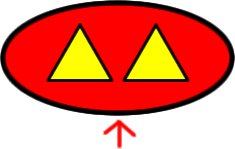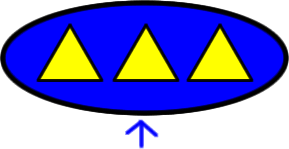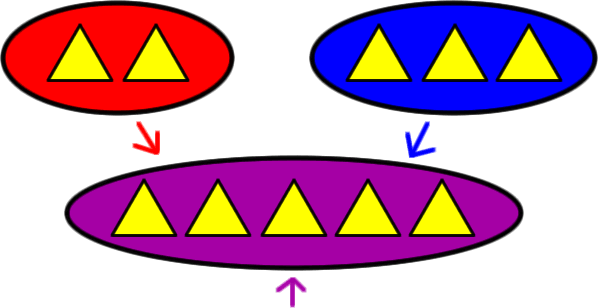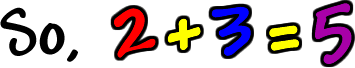Page 1 of 3
This is the very beginning of understanding how addition works.

Let's look at two sets:

 Set A Set BCount how many triangles are in Set A... There are 2! Count how many triangles are in Set B... There are 3!

Now, put the sets together into one big set:Count how many triangles are in this set...There are 5!